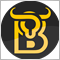# Continuing Arrows in indicator19

Hi , I need Some Help

This indicator is Called TCCI and it's Using iMA Function,

the Problem is when i want to add UpWard Or DownWard Arrows To it , it Show's Continuing Arrows! ( For Example the Shows DownWard Arrow in Every Candle until the Signal Changes To Upward )

i want it to show arrows only at the first Candle of Signal change!

can anybody help me ?

```#property copyright "Copyright © 2008, 2hrfx.com"

#property indicator_chart_window
#property indicator_buffers 3

#property indicator_color1 Red
#property indicator_width1 3

#property indicator_color2 ForestGreen
#property indicator_width2 3

#property indicator_color3 Red
#property indicator_width3 3

extern int Price = 0;

extern int Length = 65;
extern int Displace = 0;
extern int Filter = 0;
extern int Color = 1;
extern int ColorBarBack = 0;
extern double Deviation = 0.0;
extern int BarsToDraw=1000;

double MABuffer[];
double UpBuffer[];
double DnBuffer[];
double trend[];
double alfa[];
int i;
int Phase;
int Len;
int Cycle = 4;
double Coeff;
double beta;
double t;
double sum;
double Weight;
double g;
double pi = 3.1415926535;

datetime bartime;
#define under 1
#define over  2
int prevdir=0;
int currdir=0;

int init() {
IndicatorBuffers(4);
SetIndexStyle(0, DRAW_LINE);
SetIndexBuffer(0, MABuffer);

SetIndexStyle(1, DRAW_LINE);
SetIndexBuffer(1, UpBuffer);
SetIndexArrow(0,217);
SetIndexLabel(0,"UpBuffer");

SetIndexStyle(2, DRAW_LINE);
SetIndexBuffer(2, DnBuffer);
SetIndexArrow(1,218);
SetIndexLabel(1,"DnBuffer");

SetIndexBuffer(3, trend);

IndicatorDigits(MarketInfo(Symbol(), MODE_DIGITS));
string short_name = "SaneFX(" + Length + ")";
IndicatorShortName(short_name);
SetIndexLabel(0, "SaneFX");
SetIndexLabel(1, "Up");
SetIndexLabel(2, "Dn");
SetIndexShift(0, Displace);
SetIndexShift(1, Displace);
SetIndexShift(2, Displace);
SetIndexEmptyValue(0, EMPTY_VALUE);
SetIndexEmptyValue(1, EMPTY_VALUE);
SetIndexEmptyValue(2, EMPTY_VALUE);
SetIndexDrawBegin(0, Length * Cycle + Length);
SetIndexDrawBegin(1, Length * Cycle + Length);
SetIndexDrawBegin(2, Length * Cycle + Length);

Coeff = 3.0 * pi;
Phase = Length - 1;
Len = Length * Cycle + Phase;
ArrayResize(alfa, Len);
Weight = 0;
for (i = 0; i < Len - 1; i++) {
if (i <= Phase - 1) t = 1.0 * i / (Phase - 1);
else t = (i - Phase + 1) * (2.0 * Cycle - 1.0) / (Cycle * Length - 1.0) + 1.0;
beta = MathCos(pi * t);
g = 1.0 / (Coeff * t + 1.0);
if (t <= 0.5) g = 1;
alfa[i] = g * beta;
Weight += alfa[i];
}
return (0);
}

int start() {

// bar counting
if(bartime==iTime(Symbol(), 0, 0) ) return(0);
bartime=iTime(Symbol(), 0, 0) ;

int limit=BarsToDraw;
double price;

for (int i = 1; i < Length * Cycle + Length; i++) {
MABuffer[Bars - i] = 0;
UpBuffer[Bars - i] = 0;
DnBuffer[Bars - i] = 0;
}

for (int shift = limit; shift >= 0; shift--) {
sum = 0;

for (i = 0; i <= Len - 1; i++) {
if (Price == 0) price = Close[shift + i];
else {
if (Price == 1) price = Open[shift + i];
else {
if (Price == 2) price = High[shift + i];
else {
if (Price == 3) price = Low[shift + i];
else {
if (Price == 4) price = (High[shift + i] + (Low[shift + i])) / 2.0;
else {
if (Price == 5) price = (High[shift + i] + (Low[shift + i]) + (Close[shift + i])) / 3.0;
else
if (Price == 6) price = (High[shift + i] + (Low[shift + i]) + 2.0 * (Close[shift + i])) / 4.0;
}
}
}
}
}
sum += alfa[i] * price;
}
if (Weight > 0.0) MABuffer[shift] = (Deviation / 100.0 + 1.0) * sum / Weight;
if (Filter > 0)
if (MathAbs(MABuffer[shift] - (MABuffer[shift + 1])) < Filter * Point) MABuffer[shift] = MABuffer[shift + 1];

if (Color > 0) {
trend[shift] = trend[shift + 1];
if (MABuffer[shift] - (MABuffer[shift + 1]) > Filter * Point) trend[shift] = 1;
if (MABuffer[shift + 1] - MABuffer[shift] > Filter * Point) trend[shift] = -1;
if (trend[shift] > 0.0) {
UpBuffer[shift] = MABuffer[shift];
if (trend[shift + ColorBarBack] < 0.0) UpBuffer[shift + ColorBarBack] = MABuffer[shift + ColorBarBack ];
DnBuffer[shift] = EMPTY_VALUE;
if(shift==1) currdir=over;
}
if (trend[shift] < 0.0) {
DnBuffer[shift] = MABuffer[shift];
if (trend[shift + ColorBarBack] > 0.0) DnBuffer[shift + ColorBarBack] = MABuffer[shift + ColorBarBack];
UpBuffer[shift] = EMPTY_VALUE;
if(shift==1) currdir=under;
}
}
}

if(currdir!=prevdir)
{
prevdir=currdir;
{
Sleep(0);
Alert( "TCCI ",Length," "+Symbol()+" "+Hour()+":"+Minute() );
}

}

return (0);
}```

i used "DRAW_ARROW" but nothing happened!

thanks22310

Businext:
`   if(currdir!=prevdir)`

Your indicator uses global/static variables. The precondition is prevdir is the value from the previous bar. That means you can process bar zero at most once.

Either change your loop, make your variable a buffer so you can restore the variable from the previous bar, or save currdir to prevdir except on bar zero.19

William Roeder:

Your indicator uses global/static variables. The precondition is prevdir is the value from the previous bar. That means you can process bar zero at most once.

Either change your loop, make your variable a buffer so you can restore the variable from the previous bar, or save currdir to prevdir except on bar zero.

aha Thanks but Can you Please guide me more?

i didnt compeletely understand which line should i edit ?!